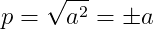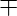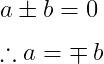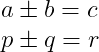# How to denote plus minus(±) symbol with latex?

You need to use the \pm command to get the latex plus-minus symbol. And you do not need to pass any argument in this command. So, take a look at this syntax below

 Properties Value Symbol Plus Minus Argument No Command \pm Example \pm → ±

For example, when you determine the square root of a number, you have to use the plus-minus sign.

\documentclass{article}
\begin{document}
$p=\sqrt{a^{2}}=\pm a$
\end{document}


Output :If you look at the program above, you can see that the\sqrt{} command is used for the square root and a² is passed as an argument.

## Plus minus symbol in text mode

You cannot use the default \pm command in direct text mode. However, with the textcomp package, you can represent plus and minus symbols in text. And the most important command for this is \textpm.

\documentclass{article}
\usepackage{textcomp}
\begin{document}
Use plus-minus symbol in text mode : \textpm
\end{document}


Output :

Use plus-minus symbol in text mode : ±

As you may know, single $or double $$dollar symbols are not used on both sides of the command in text mode. And when you use a math mode command in text mode, you use single dollars on both sides of the command. For example \documentclass{article} \begin{document} Use plus-minus symbol in text mode : \pm \end{document}  Output : Use plus-minus symbol in text mode : ± ## Minus-Plus symbol in latex Mathematically, when a character with a plus-minus sign(±) is multiplied by a minus sign(-), the plus-minus sign before that character becomes a minus-plus sign.  Properties Value Symbol Minus Plus Argument No Command \mp Example \mp →In the same way, to get the minus-plus symbol, you need to use the \mp command instead of the \pm command. For example, you notice that when a plus-minus character is moved to the opposite side of a mathematically equal, it becomes a minus-plus symbol. So, look at this LaTeX program below \documentclass{article} \begin{document} a\pm b=0 \therefore a=\mp b \end{document} Output :## Use MnSymbol, fdsymbol, boisik, and stix packages for this symbol Also, latex has multiple packages to represent this symbol. However, the interesting thing is that the same command \pm is present in each package. \documentclass{article} \usepackage{MnSymbol,fdsymbol,boisik,stix} \begin{document}$$ a \pm b = c  p \pm q = r$\$
\end{document}

Output :Hopefully, you understand how to use the plus-minus or minus plus symbol with the help of latex. And no document has been created in this tutorial, only the equation is presented in front of you.